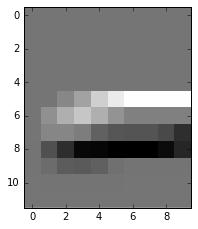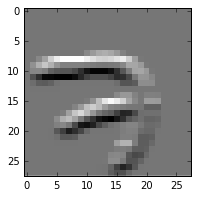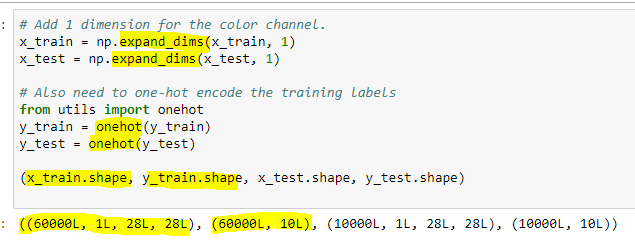# Lesson 3 discussion

Hi and thanks to @jeremy and @rachel for this wonderful class!

I’m starting the Lesson 3 lecture video, and the review of the key concepts, and I’m walking through the convolution-intro.ipynb notebook. I don’t have Tensorflow installed currently, so I followed @jeremy’s advice (which now I cannot find) and used the Keras MNIST dataset instead. Now, I’m getting strange results:

1. The number of images in the Keras dataset is different. TF has 55000, and Keras has 60000.
2. The ordering of images is different. The 0-th image in TF is number ‘7’, but the 0-th in Keras is ‘5’.
3. Most concerning is that the images demonstrating the `corrtop` details are very different.

Details:

Getting the dataset from Keras:

``````from keras.datasets import mnist
(x_train, y_train), (x_test, y_test) = mnist.load_data() # saves to /root/.keras/datasets/mnist.pkl.gz
``````

Then I assigned the `images` and `labels` variables as follows:

``````images=x_train
labels=y_train
n=len(images)
images.shape
``````

Output: `(60000, 28, 28)` (NOTE: TF was (55000, 28, 28)).

I computed the `corrtop` value using the existing code:

``````corrtop = correlate(images[inspect_idx], top)
``````

Here are the plots of the resulting `corrtop` data. The original TF versions on the left, mine on the right. Note that the numeral ‘7’ is a different index and a different sample, so the shape is different. That’s not my concern. My concern is the overall appearance. It looks like something is wrong with the filters or something.

``````plot(corrtop[dims])
``````

TF:Keras:``````plot(corrtop)
``````

TF:Keras:Can anyone shed light on why such a difference here? The only change I’ve made really is to use the Keras dataset instead of the Tensorflow dataset.

Thanks much!

Dimension ordering is different inTensorFlow compared to Theano. In TensorFlow channels come last.

I think I found a clue to this in the Lesson 3 video at: https://youtu.be/6kwQEBMandw?t=6574. Here it says that Keras expects color images, and has a channels dimension that carries that information. But the MNIST data is B/W and omits that dimension. Not accounting for this can lead to weird errors with MNIST in Keras. I think this is what my problem is.

I’ll be trying this out soon to confirm.

EDIT: I tried to make this post a reply to Lesson 3 discussion, but it didn’t seem to work. Please see that link for more context.

Well arg… I followed the advice from @jeremy in the video, and the re-dimensioned arrays look like those in the video:However, now I’m getting an error when trying to plot the array:

``````---------------------------------------------------------------------------
TypeError                                 Traceback (most recent call last)
<ipython-input-23-f1e62e015ecf> in <module>()
----> 1 plot(images[inspect_idx])

<ipython-input-15-def34cadf765> in plot(im, interp)
10 def plot(im, interp=False):
11     f = plt.figure(figsize=(3,6), frameon=True)
---> 12     plt.imshow(im, interpolation=None if interp else 'none')
13
14 plt.gray()

C:\Users\matsaleh\AppData\Local\conda\conda\envs\fastai2\lib\site-packages\matplotlib\pyplot.pyc in imshow(X, cmap, norm, aspect, interpolation, alpha, vmin, vmax, origin, extent, shape, filternorm, filterrad, imlim, resample, url, hold, data, **kwargs)
3156                         imlim=imlim, resample=resample, url=url, data=data,
-> 3157                         **kwargs)
3158     finally:
3159         ax._hold = washold

C:\Users\matsaleh\AppData\Local\conda\conda\envs\fastai2\lib\site-packages\matplotlib\__init__.pyc in inner(ax, *args, **kwargs)
1895                     warnings.warn(msg % (label_namer, func.__name__),
1896                                   RuntimeWarning, stacklevel=2)
-> 1897             return func(ax, *args, **kwargs)
1898         pre_doc = inner.__doc__
1899         if pre_doc is None:

C:\Users\matsaleh\AppData\Local\conda\conda\envs\fastai2\lib\site-packages\matplotlib\axes\_axes.pyc in imshow(self, X, cmap, norm, aspect, interpolation, alpha, vmin, vmax, origin, extent, shape, filternorm, filterrad, imlim, resample, url, **kwargs)
5122                               resample=resample, **kwargs)
5123
-> 5124         im.set_data(X)
5125         im.set_alpha(alpha)
5126         if im.get_clip_path() is None:

C:\Users\matsaleh\AppData\Local\conda\conda\envs\fastai2\lib\site-packages\matplotlib\image.pyc in set_data(self, A)
598         if (self._A.ndim not in (2, 3) or
599                 (self._A.ndim == 3 and self._A.shape[-1] not in (3, 4))):
--> 600             raise TypeError("Invalid dimensions for image data")
601
602         self._imcache = None

TypeError: Invalid dimensions for image data
``````

Seems pretty clear that matplotlib wants a 3-dim array, not 4-dim.

I thought I was on the right track, but … I still do, but there’s a missing piece to this puzzle. I’d welcome any insights from @jeremy, @rachel or anyone else at this point.

1 Like

I’m really stuck on the first section of lesson 3 due to memory issues. When I first tried model.fit() on the fc_model, it won’t run due to the trn_features array taking up almost all of my 16GB of memory. Ok, no problem, lets try fit_generator() and create a generator pulling data from the files. After wrestling with how to write a generator for a couple days, I finally got fit_generator() to work this morning… Except it didn’t work. It seems that the generator still loads the entire trn_features array into memory, causing the out of memory issue again. Can anyone help me navigate using generators on the trn_features array? Or, should I just move on to the next section (augmentation). This class seems highly sequentially structured, so I don’t want to cheat myself. However, my time for working on this class is limited (day job, six-month-old) so it’s starting to get frustrating being hung up on this one section for several weeks, all for the lack of system memory.

Here’s my generator:
def mygen(feat_arr, labels):
while True:
features = bcolz.open(feat_arr)[:]
yield (features,labels)
I am sending it the path to the feature and validation arrays that were created, as well as the labels.

This is my call to fc_model.fit_generator():
fc_model.fit_generator(train_gen, samples_per_epoch=batches.nb_sample, nb_epoch=8,
validation_data=val_gen, nb_val_samples=val_batches.nb_sample)

Here is my error message:
MemoryError: Error allocating 9232384000 bytes of device memory (out of memory).
Apply node that caused the error: GpuFromHost(maxpooling2d_input_1)
Toposort index: 9
Inputs types: [TensorType(float32, 4D)]
Inputs shapes: [(23000, 512, 14, 14)]
Inputs strides: [(401408, 784, 56, 4)]
Inputs values: [‘not shown’]
Outputs clients: [[GpuContiguous(GpuFromHost.0)]]

HINT: Re-running with most Theano optimization disabled could give you a back-trace of when this node was created. This can be done with by setting the Theano flag ‘optimizer=fast_compile’. If that does not work, Theano optimizations can be disabled with ‘optimizer=None’.
HINT: Use the Theano flag ‘exception_verbosity=high’ for a debugprint and storage map footprint of this apply node.

If it is the statefarm one then the solution is to save the predictions to a bcolz array file after each batch:

You are bottlenecking the system RAM. First save_array is using memory to save the matrices by precomputing them. Then load_array is loading them and reading actively in RAM. Upon everything you are trying to use other operations too. That is leaving no room for any kind of operations.

Here’s a fix suggestion.

1. Do not pre compute the features. If you are using GPU then just read the images and feed them to CNN. By the specified batch size only that much images will be read,computed, trained etc etc. This way You can avoid system RAM bottlenecking.

2. If you are not using GPU and have too much data just get more RAM or better hardwares if you want to proceed. Also it will be better if you somehow manage to pre compute trn_features and save it. But next time just load them. See if it helps preventing the RAM bottleneck. If not again. .get more RAM or reduce the dataset.

3. If you reduce dataset then try step 1, 2.

Thanks for your advice. Those were some of the thoughts I had. Short of upgrading my RAM, I need to figure out how to avoid pre-computing trn_features, so it doesn’t clog up my memory. If I can’t figure that out, I’ll probably just move on without completing that section of lesson 3. Thanks again!

Pre computing the `trn_features` is not needed. Just comment out the `save_array` and `load_array` functions and delete the `trn_features` code. Then use the train data to feed directly into the CNN model with a batch size. You are good to go.

I had the same problem on two different occasions using the dogscats data set.

My GPU appeared to be running out of memory (my card is only 6GB, my system is 16GB).

I solved it by reducing my batch_size. When I first started I was using batch_size=64. The first time I hit the problem, I reduced to batch_size=32. Later, when I hit it again, I reduced to batch_size=16, and have not hit the problem again.

Of course, my code runs slower. I haven’t measured it accurately, but I think it’s probably 50% slower with the smaller batches. Also these runs are all using a smaller sample set with 2000 training/500 validation images. When I get around to running the full set, I will do it in the cloud (FloydHub).

Cheers, Matt

1 Like

lesson3.ipynb: Why two (seemingly) redundant batchnorm models?

In working through the lesson3.ipynb notebook, It appears that we are creating two separate but identical models using the Vgg16bn batchnorm layers and weights:

The first one, we create here:

``````# create model with batcnorm
bn_model = Sequential(get_bn_layers(0.6))

# copy the weights from Vgg16bn

# Adjust the copied weights
for l in bn_model.layers:
if type(l)==Dense: l.set_weights(proc_wgts(l, 0.5, 0.6))

# Remove last layer and lock all the others
bn_model.pop()
for layer in bn_model.layers: layer.trainable=False

# Add linear layer (2-class) (just doing the ImageNet mapping to Kaggle dogs and cats)

# compile and fit
bn_model.fit(trn_features, trn_labels, nb_epoch=8, validation_data=(val_features, val_labels))
``````

And then we do it again here:

``````# create 2nd set of batchnorm layers
bn_layers = get_bn_layers(0.6)

# remove last layer (no lock of remaining layers?)
bn_layers.pop()

# add linear layer  (2-class) (just doing the ImageNet mapping to Kaggle dogs and cats)
bn_layers.append(Dense(2,activation='softmax'))

# create final model using conv layers from earlier (and lock everything)
final_model = Sequential(conv_layers)
for layer in final_model.layers: layer.trainable = False

# merge the 2nd batchnorm layers into the final model
for layer in bn_layers: final_model.add(layer)

# copy the weights from the 1st batchnorm model into their counterparts in the final model.
for l1,l2 in zip(bn_model.layers, bn_layers):
l2.set_weights(l1.get_weights())

# compile and fit
loss='categorical_crossentropy', metrics=['accuracy'])
final_model.fit_generator(batches, samples_per_epoch=batches.nb_sample, nb_epoch=1,
validation_data=val_batches, nb_val_samples=val_batches.nb_sample)

# more fitting and saving omitted...
``````

Why are we doing this twice? There are some differences, but they appear superficial to me. In the end, we are adding to the final model a single set of batchnorm layers pulled from the Vgg16bn model, along with their hand-picked weights.

Why not just skip the second batchnorm model and just copy the layers from the fist one into the final model?

Thanks to @jeremy or @rachel or anyone else who can shed light on this.

If pre-computing trn_features is not needed, why did we split the model into the convolutional part and the fully connected part?

My problem doesn’t arise with running the CNN, that part works just fine (trn_featues = conv_model.predict_generator(batches, batches.nb_sample). It’s when I get to the fully connected model, there’s no room left to run fc_model.fit(). The FC model is expecting data in the form of (samples, 512, 14, 14). I can’t just send the images directly to the fc_model because they are of the form (samples, 3, 224, 224) - the images themselves.

Am I off target here? I think I understand what you’re suggesting, but I haven’t been able to make that work. I suppose I could just cut the dataset in half, and just work with that, but I’d really like to work with the full set. I’ve had to craft some workarounds before, but this one has me stuck.

If [quote=“cold_fashioned, post:171, topic:186”]
The FC model is expecting data in the form of (samples, 512, 14, 14). I can’t just send the images directly to the fc_model because they are of the form (samples, 3, 224, 224) - the images themselves.
[/quote]

Is there a need to resize the images to 14, 14? If yes then just resize the images in the very first layer of model or the input layer.

I’ve a strange Result on my own dataset with Ensembling - described within the mnist Part at the end of lesson3.

any idea how i can fix this?

Here I’m using Dogs vs Cats Redux competition data.
I want to train a few layers:

``````vgg = Vgg16()
model = vgg.model
layers = model.layers

model.pop()

for layer in layers: layer.trainable = False

model.compile(optimizer=RMSprop(lr=0.001),
loss='categorical_crossentropy',
metrics=['accuracy'])

first_dense_layer = [idx for (idx, layer) in enumerate(layers) if type(layer) is Dense]
for layer in layers[first_dense_layer:]: trainable = True

batch_size = 8

train_batches = get_batches(path + 'train', shuffle=True, batch_size=batch_size)
valid_batches = get_batches(path + 'valid', shuffle=False, batch_size=batch_size)

steps_per_epoch = int(np.ceil(train_batches.n/batch_size))
validation_steps = int(np.ceil(valid_batches.n/batch_size))

model.fit_generator(train_batches,
steps_per_epoch=steps_per_epoch,
epochs=2,
validation_data=valid_batches,
validation_steps=validation_steps)
``````

When the training has been completed, I can see that the validation set accuracy is: 0.98
Then, I try to see the confusion matrix:

``````probs = model.predict_generator(valid_batches,  steps=validation_steps)
iscat = probs[:,0]
y_hat = np.round(1-iscat)
y     = valid_batches.classes

cm    = confusion_matrix(y, y_hat)
plot_confusion_matrix(cm, {'cats':0,'dogs':1})
``````

Now, from the confusion matrix, I can see the accuracy has decreased to a ~0.83
We are talking about the same dataset, how can it be possible?

Today I discovered why there is this difference …
If I compute the steps in this way:

``````steps_per_epoch = int(np.ceil(train_batches.samples/batch_size))
validation_steps = int(np.ceil(valid_batches.samples/batch_size))
``````

then the accuracy for the validation set is exactly the same that I have found during the training,~98%, of course as it should be.
I still don’t understand why I get a “wrong” accuracy if I compute steps using `n` and not `samples`. Steps per epoch and validation steps are the same (2875, 250), no matter if you use the `n` or `samples`.

Hope to have been clear …
Can anybody explain it to me?

Thanks!

@Buzz
I’m using `.n` and get the same accuracy.
But i had the same issues! I only save the weights of the Epoch with the highest `val_acc` load it afterwards and use this model to test the accuracy with:

``````score = model.evaluate(x_valid,y_valid, batch_size=batch_size)
print("%s: %.2f%%" % (model.metrics_names, score*100))
``````

i had different results here then while i trained the set. However, the issue was that i loaded my `x, y_valid` with my own data loader. I couldn’t see any difference between the loaded data, but it appears that when i loaded `x, y_valid` exactly the same way i’ve loaded my train and valid batches the results matched perfectly. Before that i was messing around with `n`and `samples` as well.

Here is how i load my images:

``````train_datagen = ImageDataGenerator(
rescale = 1./255,
width_shift_range=0.08,
height_shift_range=0.05,
horizontal_flip=True,
zoom_range=0.1,
fill_mode='constant')

test_datagen = ImageDataGenerator(
rescale=1./255)

train_generator = train_datagen.flow_from_directory(
path+'train',
target_size=(299, 299),
batch_size=batch_size,
class_mode='categorical')

validation_generator = test_datagen.flow_from_directory(
path+'valid',
target_size=(299, 299),
batch_size=64,
class_mode='categorical')

steps_per_epoch = int(np.ceil(train_generator.n/batch_size))
validation_steps = int(np.ceil(validation_generator.n/batch_size))
``````

and then

``````#--- Lädt die Validation Daten als arrays ein.
gen_val = ImageDataGenerator(
rescale=1./255)

gen = gen_val.flow_from_directory(
path+'valid',
target_size=(299, 299),
batch_size=1,
shuffle=False)

x_valid = np.concatenate([gen.next() for i in range(gen.n)])

y_valid = np.concatenate([gen.next() for i in range(gen.n)])
``````

I think that may is your problem. Otherwise i’m interested in the reason as well.

I am trying to run convolution-intro.ipynb notebook , I found `data = np.load("MNIST_data/train.npz")` , can I know from where I can get data in `.npz` format , couldn’t find it on kaggle , Thank you

@RazZzoR
You are right, `.n` or `.samples` doesn’t matter.
Today i spent some time on this and I noticed that after you have trained the model:

``````model.fit_generator(train_batches,
steps_per_epoch=steps_per_epoch,
epochs=2,
validation_data=valid_batches,
validation_steps=validation_steps)
``````

If you want to check the accuracy on the validation set (just to be clear, I’m doing this just to be sure I get a correct result by applying the following code on unlabeled data), you have to grab batches of data from the validation folder once again:

``````valid_batches = get_batches(path + 'valid', shuffle=False, batch_size=batch_size)
validation_steps = int(np.ceil(valid_batches.samples/batch_size))
print(validation_steps)
``````

This was the missing piece.
After that, you can see that the validation set accuracy is exactly the same you get from the training step (of course as it should be).

``````probs = model.predict_generator(valid_batches, steps=validation_steps)
iscat = probs[:,0]
y_hat = np.round(1-iscat)
y     = valid_batches.classes

cm    = confusion_matrix(y, y_hat)
plot_confusion_matrix(cm, {'cats':0,'dogs':1})``````

Post augmentation in lesson 3, I am running the below line of code -

``````for layer in conv_model.layers: layer.trainable = False
# Look how easy it is to connect two models together!
``````

Getting the below error. I have set the data format as K.set_image_dim_ordering(‘th’). I also check the get_batches image shape using code

``````for obj in val_batches:
print(obj)
ob = obj
break
ob.shape
output: (3, 224, 224)
``````

Error :
/home/ubuntu/anaconda2/lib/python2.7/site-packages/keras/layers/pooling.pyc in compute_output_shape(self, input_shape)
129 def compute_output_shape(self, input_shape):
130 if self.data_format == ‘channels_first’:
–> 131 rows = input_shape
132 cols = input_shape
133 elif self.data_format == ‘channels_last’:

IndexError: tuple index out of range

What’s wrong?